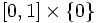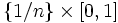# Comb space

This article describes a standard counterexample to some plausible but false implications. In other words, it lists a pathology that may be useful to keep in mind to avoid pitfalls in proofs
View other standard counterexamples in topology

## Definition

The comb space is defined as the following subset of$\R^2$ with the subspace topology: It is the union of$[0,1] \times \{ 0 \}$,$\{ 0 \} \times [0,1]$, and all line segments of the form$\{ 1/n \} \times [0,1]$ where$n$ varies over the positive integers.

## Topological space properties

### Properties it does satisfy

• Closed sub-Euclidean space
• Metrizable space
• Contractible space
• SDR-contractible space, viz it admits the origin as a strong deformation retract, via the homotopy that first collapses all the vertical line segments to the$x$-axis, and then collapses the entire$x$-axis to the origin.

### Properties it does not satisfy

• Suddenly contractible space: There is no contracting homotopy that is a homeomorphism for all$t < 1$
• Everywhere SDR-contractible space: In particular, the point$(0,1)$ is not a strong deformation retract
• Locally path-connected space: The neighbourhood of$(0,1)$ has infinitely many path components.